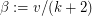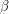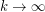Importance: Medium ✭✭
 Author(s): Rüdinger, Andreas
 Subject: Graph Theory » Basic Graph Theory
 Keywords: polyhedral graphs, distribution
Problem   Consider the set of all topologically inequivalent polyhedra withedges. Define a form parameter for a polyhedron aswhereis the number of vertices. What is the distribution offor?
Consider the set of all topologically inequivalent polyhedra on a sphere with k edges (i.e. polyhedral graphs, Sloan Sequence A002840 ). Due to duality the distribution of the form parameteris symmetric about. Now a natural question is whether the distribution of beta tends to a limiting distribution when the number of edges tends to infinity. Is there any nontrivial limit theorem by means of rescaling? Some numerical values can be found on Counting Polyhedra suggesting that the distribution concentrates around.